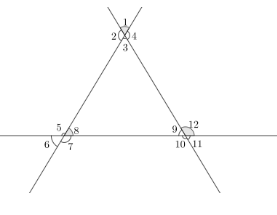Courses
Courses for Kids
Free study material
Free LIVE classes
MoreLIVE
Join Vedantu’s FREE Mastercalss

# Find each linear pair and vertically opposite angles that are present in the given figure?Verified
334.8k+ views
Hint: We recall the definition of linear pair angles and vertically opposite angles in order to find them. We check linear pair angles first at each point of intersection of lines and note them. Later, we find the vertically opposite angles at each point of intersection of lines and note them.

We have a figure given that has three lines intersecting each other. We have 12 angles namely 1,2,3,….12. We need to find the angles that are linear pairs and vertically opposite angles.
Before that we recall what are linear pair angles and vertically opposite angles.
Linear pair angles are two angles that lie on the same line and the sum of those two angles are supplementary (sum of angles = ${{180}^{o}}$ ).
Vertically opposite angles are two angles that are opposite to each other at the point where two lines intersect each other. Vertically opposite angles are always equal.
Let us first find the linear pair angles in the problem.
We can see that $\angle 1$ and $\angle 4$ lie on the same and the sum of those two angles is ${{180}^{o}}$ . So, we can say $\angle 1$ and $\angle 4$ are linear pairs.
We can see that $\angle 1$ and $\angle 2$ lie on same and sum of those two angles is ${{180}^{o}}$ . So, we can say $\angle 1$ and $\angle 2$ are linear pair.
We can see that $\angle 2$ and $\angle 3$ lie on same and sum of those two angles is ${{180}^{o}}$ . So, we can say $\angle 2$ and $\angle 3$ are linear pair.
We can see that $\angle 4$ and $\angle 3$ lie on same and sum of those two angles is ${{180}^{o}}$ . So, we can say $\angle 4$ and $\angle 3$ are linear pair.
We can see that $\angle 5$ and $\angle 6$ lie on same and sum of those two angles is ${{180}^{o}}$ . So, we can say $\angle 5$ and $\angle 6$ are linear pair.
We can see that $\angle 5$ and $\angle 8$ lie on same and sum of those two angles is ${{180}^{o}}$ . So, we can say $\angle 5$ and $\angle 8$ are linear pair.
We can see that $\angle 6$ and $\angle 7$ lie on same and sum of those two angles is ${{180}^{o}}$ . So, we can say $\angle 6$ and $\angle 7$ are linear pair.
We can see that $\angle 7$ and $\angle 8$ lie on same and sum of those two angles is ${{180}^{o}}$ . So, we can say $\angle 7$ and $\angle 8$ are linear pair.
We can see that $\angle 9$ and $\angle 10$ lie on same and sum of those two angles is ${{180}^{o}}$ . So, we can say $\angle 9$ and $\angle 10$ are linear pair.
We can see that $\angle 9$ and $\angle 12$ lie on same and sum of those two angles is ${{180}^{o}}$ . So, we can say $\angle 9$ and $\angle 12$ are linear pair.
We can see that $\angle 10$ and $\angle 11$ lie on same and sum of those two angles is ${{180}^{o}}$ . So, we can say $\angle 10$ and $\angle 11$ are linear pair.
We can see that $\angle 11$ and $\angle 12$ lie on same and sum of those two angles is ${{180}^{o}}$ . So, we can say $\angle 11$ and $\angle 12$ are linear pair.
Now, we find the vertically opposite angles.
We can see that $\angle 1$ and $\angle 3$ lie opposite to each other at the point of intersection of lines. So, we can say $\angle 1$ and $\angle 3$ are vertically opposite angles.
We can see that $\angle 2$ and $\angle 4$ lie opposite to each other at the point of intersection of lines. So, we can say $\angle 2$ and $\angle 4$ are vertically opposite angles.
We can see that $\angle 5$ and $\angle 7$ lie opposite to each other at the point of intersection of lines. So, we can say $\angle 5$ and $\angle 7$ are vertically opposite angles.
We can see that $\angle 6$ and $\angle 8$ lie opposite to each other at the point of intersection of lines. So, we can say $\angle 6$ and n$\angle 8$ are vertically opposite angles.
We can see that $\angle 9$ and $\angle 11$ lie opposite to each other at the point of intersection of lines. So, we can say $\angle 9$ and $\angle 11$ are vertically opposite angles.
We can see that $\angle 10$ and $\angle 12$ lie opposite to each other at the point of intersection of lines. So, we can say $\angle 10$ and $\angle 12$ are vertically opposite angles.
The linear pair angles are:
1) $\angle 1$ and $\angle 4$
2) $\angle 1$ and $\angle 2$
3) $\angle 2$ and $\angle 3$
4) $\angle 4$ and $\angle 3$
5) $\angle 5$ and $\angle 6$
6) $\angle 5$ and $\angle 8$
7) $\angle 6$ and $\angle 7$
8) $\angle 7$ and $\angle 8$
9) $\angle 9$ and $\angle 10$
10) $\angle 9$ and $\angle 12$
11) $\angle 10$ and $\angle 11$
12) $\angle 11$ and $\angle 12$
The vertically opposite angles are:
1) $\angle 1$ and $\angle 3$
2) $\angle 2$ and $\angle 4$
3) $\angle 5$ and $\angle 7$
4) $\angle 6$ and $\angle 8$
5) $\angle 9$ and $\angle 11$
6) $\angle 10$ and $\angle 12$

Note: We should not confuse the definition of linear pair angles with vertically opposite angles. We always need to make sure that linear pair angles lie on a line sum is ${{180}^{o}}$. We also need to make sure that we find vertically opposite angles without any confusion.
Last updated date: 26th Sep 2023
Total views: 334.8k
Views today: 7.34k